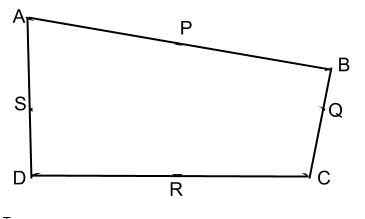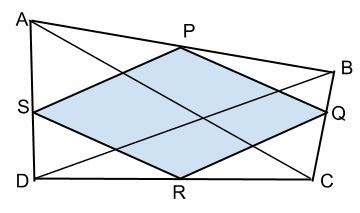Courses

# Theory - To show that the figure obtained by joining the mid-points of consecutive sides, Math Class 9 Notes | EduRev

## Mathematics (Maths) Class 9

Created by: Indu Gupta

## Class 9 : Theory - To show that the figure obtained by joining the mid-points of consecutive sides, Math Class 9 Notes | EduRev

The document Theory - To show that the figure obtained by joining the mid-points of consecutive sides, Math Class 9 Notes | EduRev is a part of the Class 9 Course Mathematics (Maths) Class 9.
All you need of Class 9 at this link: Class 9

Objective:

To show that the figure obtained by joining the mid-points of consecutive sides of the quadrilateral is a parallelogram.

Parallelogram:

1. A parallelogram is a simple quadrilateral with two pairs of parallel sides.

2. The opposite or facing sides of a parallelogram are of equal length.

Proof:

In quadrilateral ABCD points P, Q, R, S are midpoints of side AB, BC, CD and AD respectively.To prove :

PS || QR  and SR || PQ. i.e. Quadrilateral PQRS is a parallelogram

Proof:

1. Draw diagonal BD.

2. As PS is the midsegment of ▲ ABD, we can say that PS || BD.

3. As QR is the midsegment of ▲ BCD, we can say that QR || BD.

4. ∵ PS || BD and QR || BD by transitivity, we can say that PS || QR.

5. Now draw diagonal AC.

6. As SR is the midsegment of ▲ ACD, we can say that SR || AC.

7. As PQ is the midsegment of ▲ ABC, we can say that PQ || AC.

8. ∵ SR || AC and PQ || AC by transitivity, we can say that SR || PQ.

9. ∵ PS || QR and SR || PQ, ∴ quadrilateral PQRS is a parallelogram (by definition).

## Mathematics (Maths) Class 9

192 videos|230 docs|82 tests

,

,

,

,

,

,

,

,

,

,

,

,

,

,

,

,

,

,

,

,

,

,

,

,

;